Courses

# Multiplexers Practice Quiz - 1

## 14 Questions MCQ Test Mock Test Series - Computer Science Engg. (CSE) GATE 2020 | Multiplexers Practice Quiz - 1

Description
This mock test of Multiplexers Practice Quiz - 1 for Computer Science Engineering (CSE) helps you for every Computer Science Engineering (CSE) entrance exam. This contains 14 Multiple Choice Questions for Computer Science Engineering (CSE) Multiplexers Practice Quiz - 1 (mcq) to study with solutions a complete question bank. The solved questions answers in this Multiplexers Practice Quiz - 1 quiz give you a good mix of easy questions and tough questions. Computer Science Engineering (CSE) students definitely take this Multiplexers Practice Quiz - 1 exercise for a better result in the exam. You can find other Multiplexers Practice Quiz - 1 extra questions, long questions & short questions for Computer Science Engineering (CSE) on EduRev as well by searching above.
QUESTION: 1

### Consider the following Boolean function of four variables: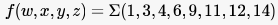The function is

Solution:

The K-map would be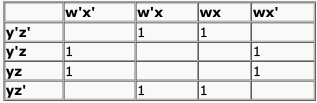So, the minimized expression would
be x'z + xz'.
So, option B.

QUESTION: 2

### Consider the following Boolean function of four variables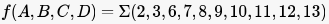The function is

Solution: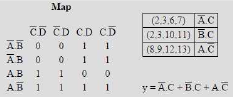QUESTION: 3

### The simplified SOP (Sum of Product) from the Boolean expression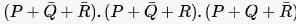1s

Solution: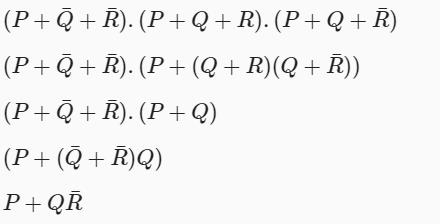QUESTION: 4

Consider the 4-to-1 multiplexer with two select lines S1 and S0 given below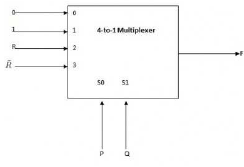The minimal sum-of-products form of the Boolean expression for the output F of the multiplexer is

Solution:

S0 and S1 are used to select the input given to be given as output.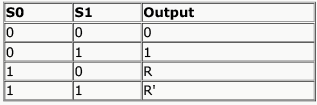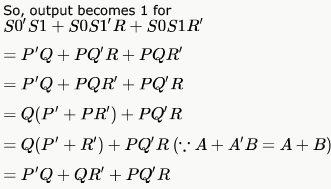QUESTION: 5

Consider the following Boolean expression for F: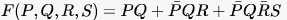The minimal sum of products form of F is

Solution: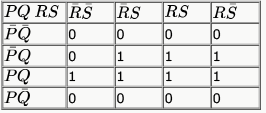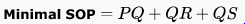Hence, option A is correct.

QUESTION: 6

Consider the following minterm expression F for :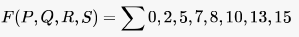The minterms 2, 7, 8 and 13 are 'do not care' terms. The minimal sum-of-products form for F is

Solution:

While putting the terms to K-map the 3rd and 4th columns are swapped so do 3rd and 4th rows. So, term 2 is going to (0,3) column instead of (0,2), 8 is going to (3,0) instead of (2,0) etc..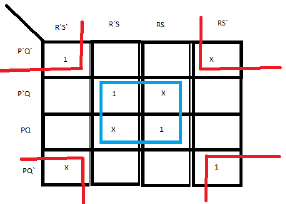Solving this k-map gives B) as the answer.

QUESTION: 7

Consider the two cascade 2 to 1 multiplexers as shown in the figure .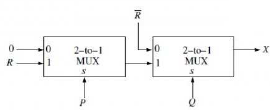The minimal sum of products form of the output X is

Solution: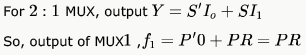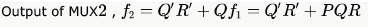QUESTION: 8

The output F of the below multiplexer circuit can be represented by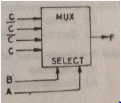Solution: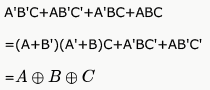QUESTION: 9

A multiplexer with a 4 - bit data select input is a

Solution:

for n bit data select inputQUESTION: 10

It is possible for an enable or strobe input to undergo an expansion of two or more MUX ICs to the digital multiplexer with the proficiency of large number of

Solution:

It is possible for an enable or strobe input to undergo an expansion of two or more MUX ICs to the digital multiplexer with the proficiency of large number of inputs.

QUESTION: 11

Consider a multiplexer with X and Y as data inputs and Z the as control input. Z = 0 selects input X, and Z = 1 selects input Y. What are the connections required to realize the 2-variable Boolean function F = T + R, without using any additional hardware?

Solution:

Z'X + ZY..
Put Z = T, X = R, Y = 1 in Z'X + ZY..
= T'R + 1*T
= (T+T') (T+R)
= T + R

QUESTION: 12

The circuit shown below implements a 2-input NOR gate using two 2  4 MUX (control signal 1 selects the upper input).
What are the values of signals x,y and z?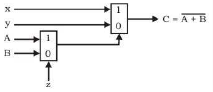Solution: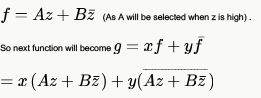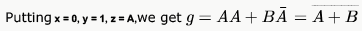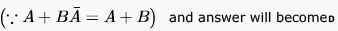QUESTION: 13

Suppose only one multiplexer and one inverter are allowed to be used to implement any Boolean function of  variables.
What is the minimum size of the multiplexer needed?

Solution:

2n - 1
We will map  variables  to select lines and  variable to input line

QUESTION: 14

The following circuit implements a two-input AND gate using two 2-1 multiplexers.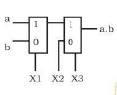What are the values of X1,X2,X3?

Solution:

F = (bX1' + aX1)X3 + X2X3'
Put X1 = b, X2 = 0, X3 = a to get F = ab.